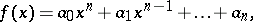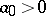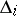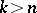# Routh-Hurwitz criterion

Jump to: navigation, search

Hurwitz criterion

A necessary and sufficient condition for all the roots of a polynomialwith real coefficients and, to have negative real parts. It consists of the following: All principal minors,, of the Hurwitz matrixare positive (cf. Minor). Hereis the matrix of orderwhose-th row has the formwhere, by definition,ifor(the Hurwitz condition or the Routh–Hurwitz condition). This criterion was obtained by A. Hurwitz  and is a generalization of the work of E.J. Routh (see Routh theorem).

A polynomialsatisfying the Hurwitz condition is called a Hurwitz polynomial, or, in applications of the Routh–Hurwitz criterion in the stability theory of oscillating systems, a stable polynomial. There are other criteria for the stability of polynomials, such as the Routh criterion, the Liénard–Chipart criterion, and methods for determining the number of real roots of a polynomial are also known.

How to Cite This Entry:
Routh-Hurwitz criterion. Encyclopedia of Mathematics. URL: http://encyclopediaofmath.org/index.php?title=Routh-Hurwitz_criterion&oldid=13913
This article was adapted from an original article by E.N. Kuz'min (originator), which appeared in Encyclopedia of Mathematics - ISBN 1402006098. See original article